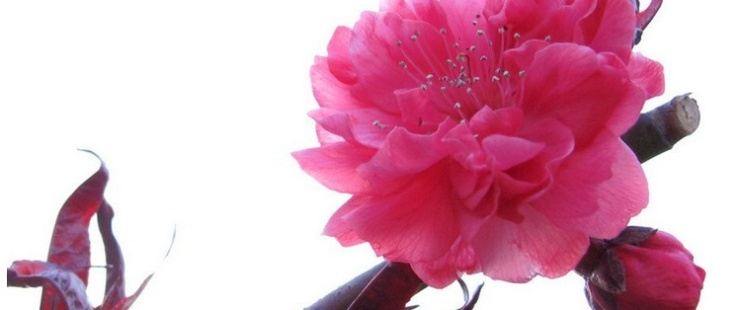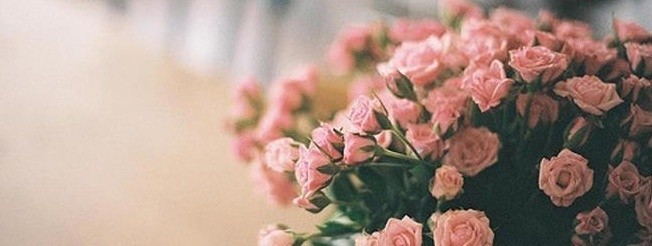|

# 如何增加桃花运风水

现代社会人们越来越多所谓的剩男剩女，你知道为什么越来越多的剩男剩女吗？这可能关系到家居的风水喔。想要增强桃花运吗？不妨随大师一起来了解下吧。1 、水晶摆放在东南有利拖拍及桃花运的增加

想拍拖的话，就要催旺位于东南的桃花位了！锦囊：“在家或office，如果床头或柜面面向东南位就最好，或者可以放粉晶、红丝带在东南位也可以！”锦囊攻略：不要放太多黑色或深色物品在桃花位。

2、玫瑰摆放西北更甜蜜并增加桃花运

已婚或正在拍拖的人，都会想关系更sweet，只要在西北是非星这个位置摆阵就可以了噢！锦囊：“今年西北位最忌放绿色，而睡房在屋子的中宫也是不好，会整天吵架。”锦囊攻略：在西北位摆放红色物品或9支去刺玫瑰。

3、公鸡摆放东南可防爱人变心及增加桃花运

整天怕男友变心的朋友有救喇，原来东南的桃花位亦可以催旺亦可以削弱的！锦囊：“在家的东南桃花位摆放木制的公鸡饰物，可以减低桃花。但记得不要放招惹坏桃花的空花瓶。”锦囊攻略：桃花亦代表人缘，如果你正在做对外的工作，如sales，就不要化解喇！

4、正东摆放双胞胎公仔可增加桃花运及旺生宝宝

想结婚或者生BB的朋友，催旺正东的喜庆位就最能帮得到你啦！锦囊：“大家只要在正东的喜庆位摆放红色、绿色物品、有果实的泥种植物或孖公仔摆设就可以了！”

锦囊攻略：正东摆放红色、绿色物品、有果实的泥种植物或者公仔摆设。

5、水种植物摆放东南增加桃花运

桃花亦代表人缘，人缘好，做任何事都自然顺利啦！锦囊：“在东南位所见的颜色越鲜艳越好，例如放红、黄和紫的物品，或放任何水种植物都有助人缘，改善人际关系。”锦囊攻略：如果想在工作中催旺人缘，就可以放些鲜色工作物件，或放盆水种植物也可以。

6、桃花要摆放在桃花位

人们常说围着桃花走几个圈就有桃花运到底是真的吗？锦囊攻略：桃花摆放在东南位。

7、向西南走有桃花运

想年初一行大运的时候就开始走桃花运，那又可以怎么做呢？锦囊：“年初一的桃花位在西南，而西南也是当日的吉位(吉时有3:00am-5:00am、5:00am-7:00am、7:00am-9:00am、11:00am-1:00pm)。”

锦囊攻略：只要大家一出家门口就向西南方走就可以啦，当然跟honey一起去拍拖，走回一个桃花运，关系就变得更甜蜜啦！

8、立春睡房摆放桃花阵最灵

摆桃花阵最好摆在自己睡房!锦囊：“所有流年桃花阵，在立春布阵的效果最好，即西历的2月4日，最有效的方法是将所有布阵工具清洁干净再用。”锦囊攻略：摆桃花阵最好摆在自己睡房！

9、屋内摆设成双

整理房间，清除过于女性化，过于破旧有菱角的东西，如果室内有仙人掌的话最好拿开，这样会帮助你早日脱离单身的生活。女人增加桃花运的风水方法

1、西北方位和中宫方位放红色

爱情的方位你了解吗?今年西北方位和中宫方位是桃花气场最强的方位了，多多在这两个方位活动，可以把卧室或者客厅安排到这个方向，另外在这两个方向多放置些红色系的饰品和家用电器，比如红色地毯，紫色水晶，电脑，加湿器等等，也可把墙壁油漆成红色，紫色或者粉色，会让你的好桃花变得更好，滥桃花变好桃花。

2、粉红色饰物留住可能会失去的爱情

你和爱人之间是否有解不开的心结，感觉他要离开你呢，这个时候应该怎么办呢，你一定有很多饰品，比如耳钉，耳环，手链，化妆盒，化妆包，可以将它们都换成粉红色的，在风水学里面，这些粉红色饰物会帮助你留住可能会失去的爱情。

3、增加圆形的家居和饰物

你们之间平平淡淡吗，你们之间互相厌倦吗，日子千篇一律的重复，爱情也越来越没有激情，这个时候你应该在房间里增加圆形的家居和饰物，比如厨房的桌子可以换成圆形的，音响，手表都可以选用圆形的，圆这个形状会增加你们爱情的凝结力，让你们的爱情更有生机。让那些有棱角的东西离你们远远的。

4、一盆水培的植物或是四季小盆栽

今年滥桃花的女孩子可不少，有滥桃花的女生们可以在自己的房间中放置一盆水培的植物或是四季小盆栽，记得水培植物要勤换水，这样会带来新鲜的气息，这是一个改变滥桃花的好方法，并且可以减少口舌，增进感情。

5、绿色的饰品

已经有爱人的女生们，是否三天两头和情人闹别扭，担心和伴侣相处不好呢，如果有这方面的问题，试试在约会的时候穿上绿色的衣服，或是带着绿色的饰品，并在布置家里的时候用绿色或者蓝色的床单，会让你和伴侣之间的关系变得和谐。

6、屋内摆设成双对

怎么会让桃花变好?扔掉旧情人送你的礼物以及他的东西吧，销毁掉这些东西，会增进你现在的桃花，大有重新开始的意味。把房间内单人的画像、海报取下来，房间里的摆设比如床头柜，音响，台灯尽量要成双成对。整理房间，清除过于女性化，过于破旧有菱角的东西，如果室内有仙人掌的话最好拿开，这样会帮助你早日脱离单身的生活。

7、不要在床头放花，卧室中不要用黑色装点!

已有情人的女生们怕自己的爱人脚踏两只船吗，如果出现这样感情危机的女生要注意千万不要在床头放花，这样会对你们之间的感情产生不好的影响，加快对方的背叛，切忌卧室中不要用黑色装点，要用红色，紫色，粉色，绿色，蓝色这几种颜色去装点。

8、不要让你的床离窗户太近，不要在床前放置大镜子!

桃花过旺或是有烂桃花的女生们有出轨或者婚外情的危险，要怎么在风水方面避免这些情况的发生呢，首先要注意不要让你的床离窗户太近，窗外代表着外界，床代表着你们的恋情，如果离窗子太近，则代表你们的爱情有危险。不要在床前放置很大的镜子，植物要选一些大叶的植物，切忌仙人掌。

9、粉水晶，水晶苹果，碎水晶开运袋

再介绍一种开桃花的好东东，粉水晶，很多明星都用粉水晶来旺桃花，你可以佩戴粉水晶的手链，项链，也可以在自己的卧室中放置粉红色的水晶球或者水晶苹果来装饰，更可以用碎水晶做成一个开运袋，随身携带，睡觉的时候放在枕边，这会大大的改变你的桃花运，是很值得一试的好方法。

10、要相信感情，憧憬美好的爱情，不要消极

其实人自己本身也是一种风水呢，要记得时时刻刻保持积极乐观的心态，要相信感情，憧憬美好的爱情，不要消极，不要压抑，这样乐观的你再加上上面那么多改变桃花的好方法，一定会让你很快找到称心如意的另一半

增加桃花运的卧室风水秘诀

想要制造浪漫的气氛其实很简单，现在告诉你一些家居布置摆放的小方法，可以提升自己的浪漫气息！还会让你的感情甜甜蜜蜜的唷！ 秘诀一放一杯水在床头可降火气. ? 一个人如果长期无法睡好，就一定会火气大，一般来说，火气大就容易口臭，而一开口就有异味的人，通常是没有迷人的魅力的。在传统的风水中，认为睡不好是头部或床头的火性能量太高，让自己的脑袋和神经无法冷却下来，才会不睡不着，所以你可能在你的床头放一杯水（要冷水不可以用热水），还有最好用磁杯或陶杯来盛水。 ? 秘诀二用聚光灯及花来增加你的美感 ?在家里的花瓶的上方装一盏聚光灯，当你心情烦闷，静不下心来时，去集中自己的精神去接受灯光下花的美感讯息，让聚光灯下的强烈美感来取代你神上的不良讯息，自然你就会拥有好心情，和美丽的心，想拥有爱情的人，不妨先从拥有美感下手。 ? 秘诀三（露骨无情煞）的化解 ? 家中有许多冷硬突出和铁铝窗架，就是它的筋骨，你想想如果你每天都面对这样的生活环境，都会让自己的心柔软不下来，相信你的爱情也会因此而殆尽。可以在这些地方加装窗帘（窗帘的效果比摆叶窗好）或摆几盆柔和的植物放置于上，这样的话你的柔软心性会增加，如此一来便可增加你的温柔性情，及有好的姻缘出现。 ? 秘诀四唤醒你的（动情激素） ? 红、橙、黄之类的颜色，对人的情感和活力，是最富刺激和催化功能，你可以在桌上放逼些暖色系的摆设，挂张暖色系的画，穿暖色系的衣服，只要你能在家中安排这些催化爱情能量的（色彩讯息），你身上的（爱情讯息）永远都不会退化衰老，你就永远都有机会和人家来电。 ? 秘诀五增加你的桃花运 ? 你可以在你的床头放着一个花瓶，在花瓶上插上桃花三株，再在睡前说出自己的姓名及你喜欢的人名字，就这样持续七天，你会有意想不到的效果，如果还没有特定对象的人，也可以增加你桃花的机会唷。

`声明：本文由入驻焦点开放平台的作者撰写，除焦点官方账号外，观点仅代表作者本人，不代表焦点立场错误信息举报电话： 400-099-0099，邮箱：jubao@vip.sohu.com，或点此进行意见反馈，或点此进行举报投诉。`A B C D E F G H J K L M N P Q R S T W X Y Z
A - B - C - D - E
• A
• 鞍山
• 安庆
• 安阳
• 安顺
• 安康
• 澳门
• B
• 北京
• 保定
• 包头
• 巴彦淖尔
• 本溪
• 蚌埠
• 亳州
• 滨州
• 北海
• 百色
• 巴中
• 毕节
• 保山
• 宝鸡
• 白银
• 巴州
• C
• 承德
• 沧州
• 长治
• 赤峰
• 朝阳
• 长春
• 常州
• 滁州
• 池州
• 长沙
• 常德
• 郴州
• 潮州
• 崇左
• 重庆
• 成都
• 楚雄
• 昌都
• 慈溪
• 常熟
• D
• 大同
• 大连
• 丹东
• 大庆
• 东营
• 德州
• 东莞
• 德阳
• 达州
• 大理
• 德宏
• 定西
• 儋州
• 东平
• E
• 鄂尔多斯
• 鄂州
• 恩施
F - G - H - I - J
• F
• 抚顺
• 阜新
• 阜阳
• 福州
• 抚州
• 佛山
• 防城港
• G
• 赣州
• 广州
• 桂林
• 贵港
• 广元
• 广安
• 贵阳
• 固原
• H
• 邯郸
• 衡水
• 呼和浩特
• 呼伦贝尔
• 葫芦岛
• 哈尔滨
• 黑河
• 淮安
• 杭州
• 湖州
• 合肥
• 淮南
• 淮北
• 黄山
• 菏泽
• 鹤壁
• 黄石
• 黄冈
• 衡阳
• 怀化
• 惠州
• 河源
• 贺州
• 河池
• 海口
• 红河
• 汉中
• 海东
• 怀来
• I
• J
• 晋中
• 锦州
• 吉林
• 鸡西
• 佳木斯
• 嘉兴
• 金华
• 景德镇
• 九江
• 吉安
• 济南
• 济宁
• 焦作
• 荆门
• 荆州
• 江门
• 揭阳
• 金昌
• 酒泉
• 嘉峪关
K - L - M - N - P
• K
• 开封
• 昆明
• 昆山
• L
• 廊坊
• 临汾
• 辽阳
• 连云港
• 丽水
• 六安
• 龙岩
• 莱芜
• 临沂
• 聊城
• 洛阳
• 漯河
• 娄底
• 柳州
• 来宾
• 泸州
• 乐山
• 六盘水
• 丽江
• 临沧
• 拉萨
• 林芝
• 兰州
• 陇南
• M
• 牡丹江
• 马鞍山
• 茂名
• 梅州
• 绵阳
• 眉山
• N
• 南京
• 南通
• 宁波
• 南平
• 宁德
• 南昌
• 南阳
• 南宁
• 内江
• 南充
• P
• 盘锦
• 莆田
• 平顶山
• 濮阳
• 攀枝花
• 普洱
• 平凉
Q - R - S - T - W
• Q
• 秦皇岛
• 齐齐哈尔
• 衢州
• 泉州
• 青岛
• 清远
• 钦州
• 黔南
• 曲靖
• 庆阳
• R
• 日照
• 日喀则
• S
• 石家庄
• 沈阳
• 双鸭山
• 绥化
• 上海
• 苏州
• 宿迁
• 绍兴
• 宿州
• 三明
• 上饶
• 三门峡
• 商丘
• 十堰
• 随州
• 邵阳
• 韶关
• 深圳
• 汕头
• 汕尾
• 三亚
• 三沙
• 遂宁
• 山南
• 商洛
• 石嘴山
• T
• 天津
• 唐山
• 太原
• 通辽
• 铁岭
• 泰州
• 台州
• 铜陵
• 泰安
• 铜仁
• 铜川
• 天水
• 天门
• W
• 乌海
• 乌兰察布
• 无锡
• 温州
• 芜湖
• 潍坊
• 威海
• 武汉
• 梧州
• 渭南
• 武威
• 吴忠
• 乌鲁木齐
X - Y - Z
• X
• 邢台
• 徐州
• 宣城
• 厦门
• 新乡
• 许昌
• 信阳
• 襄阳
• 孝感
• 咸宁
• 湘潭
• 湘西
• 西双版纳
• 西安
• 咸阳
• 西宁
• 仙桃
• 西昌
• Y
• 运城
• 营口
• 盐城
• 扬州
• 鹰潭
• 宜春
• 烟台
• 宜昌
• 岳阳
• 益阳
• 永州
• 阳江
• 云浮
• 玉林
• 宜宾
• 雅安
• 玉溪
• 延安
• 榆林
• 银川
• Z
• 张家口
• 镇江
• 舟山
• 漳州
• 淄博
• 枣庄
• 郑州
• 周口
• 驻马店
• 株洲
• 张家界
• 珠海
• 湛江
• 肇庆
• 中山
• 自贡
• 资阳
• 遵义
• 昭通
• 张掖
• 中卫

1室1厅1厨1卫1阳台

1
2
3
4
5

0
1
2

1

1

0
1
2
3报名成功，资料已提交审核A B C D E F G H J K L M N P Q R S T W X Y Z
A - B - C - D - E
• A
• 鞍山
• 安庆
• 安阳
• 安顺
• 安康
• 澳门
• B
• 北京
• 保定
• 包头
• 巴彦淖尔
• 本溪
• 蚌埠
• 亳州
• 滨州
• 北海
• 百色
• 巴中
• 毕节
• 保山
• 宝鸡
• 白银
• 巴州
• C
• 承德
• 沧州
• 长治
• 赤峰
• 朝阳
• 长春
• 常州
• 滁州
• 池州
• 长沙
• 常德
• 郴州
• 潮州
• 崇左
• 重庆
• 成都
• 楚雄
• 昌都
• 慈溪
• 常熟
• D
• 大同
• 大连
• 丹东
• 大庆
• 东营
• 德州
• 东莞
• 德阳
• 达州
• 大理
• 德宏
• 定西
• 儋州
• 东平
• E
• 鄂尔多斯
• 鄂州
• 恩施
F - G - H - I - J
• F
• 抚顺
• 阜新
• 阜阳
• 福州
• 抚州
• 佛山
• 防城港
• G
• 赣州
• 广州
• 桂林
• 贵港
• 广元
• 广安
• 贵阳
• 固原
• H
• 邯郸
• 衡水
• 呼和浩特
• 呼伦贝尔
• 葫芦岛
• 哈尔滨
• 黑河
• 淮安
• 杭州
• 湖州
• 合肥
• 淮南
• 淮北
• 黄山
• 菏泽
• 鹤壁
• 黄石
• 黄冈
• 衡阳
• 怀化
• 惠州
• 河源
• 贺州
• 河池
• 海口
• 红河
• 汉中
• 海东
• 怀来
• I
• J
• 晋中
• 锦州
• 吉林
• 鸡西
• 佳木斯
• 嘉兴
• 金华
• 景德镇
• 九江
• 吉安
• 济南
• 济宁
• 焦作
• 荆门
• 荆州
• 江门
• 揭阳
• 金昌
• 酒泉
• 嘉峪关
K - L - M - N - P
• K
• 开封
• 昆明
• 昆山
• L
• 廊坊
• 临汾
• 辽阳
• 连云港
• 丽水
• 六安
• 龙岩
• 莱芜
• 临沂
• 聊城
• 洛阳
• 漯河
• 娄底
• 柳州
• 来宾
• 泸州
• 乐山
• 六盘水
• 丽江
• 临沧
• 拉萨
• 林芝
• 兰州
• 陇南
• M
• 牡丹江
• 马鞍山
• 茂名
• 梅州
• 绵阳
• 眉山
• N
• 南京
• 南通
• 宁波
• 南平
• 宁德
• 南昌
• 南阳
• 南宁
• 内江
• 南充
• P
• 盘锦
• 莆田
• 平顶山
• 濮阳
• 攀枝花
• 普洱
• 平凉
Q - R - S - T - W
• Q
• 秦皇岛
• 齐齐哈尔
• 衢州
• 泉州
• 青岛
• 清远
• 钦州
• 黔南
• 曲靖
• 庆阳
• R
• 日照
• 日喀则
• S
• 石家庄
• 沈阳
• 双鸭山
• 绥化
• 上海
• 苏州
• 宿迁
• 绍兴
• 宿州
• 三明
• 上饶
• 三门峡
• 商丘
• 十堰
• 随州
• 邵阳
• 韶关
• 深圳
• 汕头
• 汕尾
• 三亚
• 三沙
• 遂宁
• 山南
• 商洛
• 石嘴山
• T
• 天津
• 唐山
• 太原
• 通辽
• 铁岭
• 泰州
• 台州
• 铜陵
• 泰安
• 铜仁
• 铜川
• 天水
• 天门
• W
• 乌海
• 乌兰察布
• 无锡
• 温州
• 芜湖
• 潍坊
• 威海
• 武汉
• 梧州
• 渭南
• 武威
• 吴忠
• 乌鲁木齐
X - Y - Z
• X
• 邢台
• 徐州
• 宣城
• 厦门
• 新乡
• 许昌
• 信阳
• 襄阳
• 孝感
• 咸宁
• 湘潭
• 湘西
• 西双版纳
• 西安
• 咸阳
• 西宁
• 仙桃
• 西昌
• Y
• 运城
• 营口
• 盐城
• 扬州
• 鹰潭
• 宜春
• 烟台
• 宜昌
• 岳阳
• 益阳
• 永州
• 阳江
• 云浮
• 玉林
• 宜宾
• 雅安
• 玉溪
• 延安
• 榆林
• 银川
• Z
• 张家口
• 镇江
• 舟山
• 漳州
• 淄博
• 枣庄
• 郑州
• 周口
• 驻马店
• 株洲
• 张家界
• 珠海
• 湛江
• 肇庆
• 中山
• 自贡
• 资阳
• 遵义
• 昭通
• 张掖
• 中卫• 手机• 分享
• 设计
免费设计
• 计算器
装修计算器
• 入驻
合作入驻
• 联系
联系我们
• 置顶
返回顶部